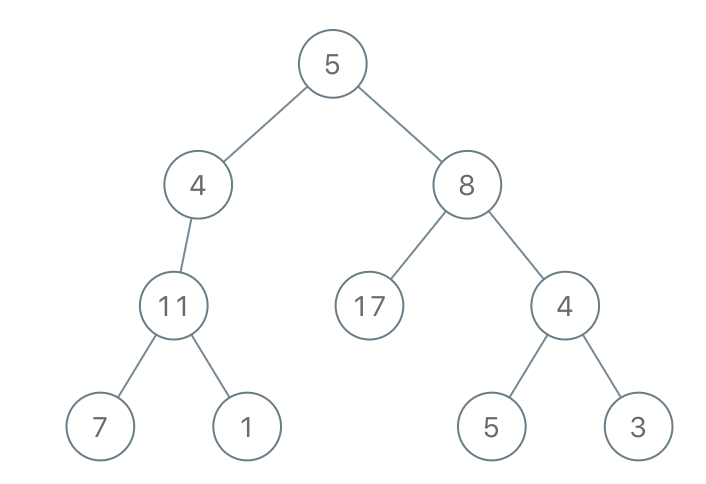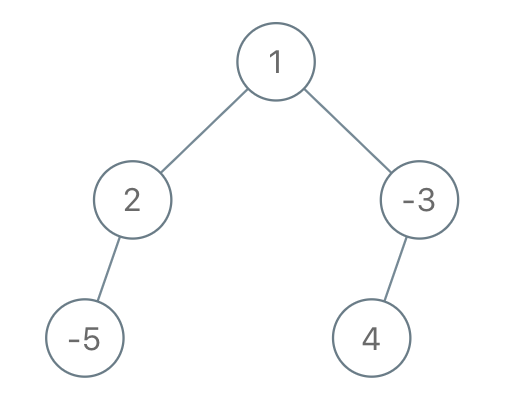## Algorithm

Problem Name: 1080. Insufficient Nodes in Root to Leaf Paths

Given the `root` of a binary tree and an integer `limit`, delete all insufficient nodes in the tree simultaneously, and return the root of the resulting binary tree.

A node is insufficient if every root to leaf path intersecting this node has a sum strictly less than `limit`.

A leaf is a node with no children.

Example 1:```Input: root = [1,2,3,4,-99,-99,7,8,9,-99,-99,12,13,-99,14], limit = 1
Output: [1,2,3,4,null,null,7,8,9,null,14]
```

Example 2:```Input: root = [5,4,8,11,null,17,4,7,1,null,null,5,3], limit = 22
Output: [5,4,8,11,null,17,4,7,null,null,null,5]
```

Example 3:```Input: root = [1,2,-3,-5,null,4,null], limit = -1
Output: [1,null,-3,4]
```

Constraints:

• The number of nodes in the tree is in the range `[1, 5000]`.
• `-105 <= Node.val <= 105`
• `-109 <= limit <= 109`

## Code Examples

### #1 Code Example with Java Programming

```Code - Java Programming```

``````
class Solution {
public TreeNode sufficientSubset(TreeNode root, int limit) {
if (root == null) {
return null;
}
if (root.left == null && root.right == null) {
return root.val < limit ? null : root;
}
root.left = sufficientSubset(root.left, limit - root.val);
root.right = sufficientSubset(root.right, limit - root.val);
return root.left == root.right ? null : root;
}
}
``````
Copy The Code &

Input

cmd
root = [1,2,3,4,-99,-99,7,8,9,-99,-99,12,13,-99,14], limit = 1

Output

cmd
[1,2,3,4,null,null,7,8,9,null,14]

### #2 Code Example with Python Programming

```Code - Python Programming```

``````
class Solution:
def sufficientSubset(self, root: TreeNode, limit: int) -> TreeNode:
if root.left == root.right is None:
return None if root.val < limit else root
if root.left:
root.left = self.sufficientSubset(root.left, limit - root.val)
if root.right:
root.right = self.sufficientSubset(root.right, limit - root.val)
return root if root.left or root.right else None
``````
Copy The Code &

Input

cmd
root = [1,2,3,4,-99,-99,7,8,9,-99,-99,12,13,-99,14], limit = 1

Output

cmd
[1,2,3,4,null,null,7,8,9,null,14]